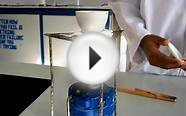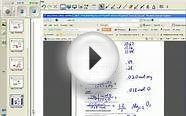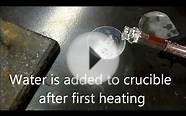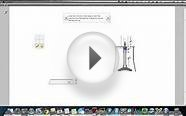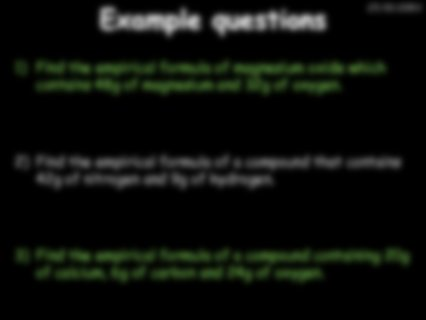# Empirical formula of Magnesium oxide

Formula of a Compound I: Magnesium and Oxygen

## Overview

• In this experiment, you precisely weigh a sample of magnesium metal, and then heat the sample in the air. Magnesium metal reacts with the oxygen (O2) of the air to form magnesium oxide. Mg + O2 ® magnesium oxide

After the magnesium sample has reacted completely, you determine the mass of magnesium oxide product. From these two masses, you calculate the percentage composition of magnesium oxide.

## Calculations

• Suppose the data below were recorded for this experiment (see Page 33, Section I).
 Mass of magnesium 0.3397 g Mass of crucible, cover, beaker 45.7324 g Mass of crucible, cover, beaker, product after first heating 46.3105 g after second heating 46.2995 g after third heating (if performed) 46.2991 g
• Note that the experiment calls for you to determine in a small beaker the initial mass of magnesium metal taken. The mass of magnesium recorded for Page 33, Section I, part (a) should be only the mass of the magnesium metal itself (not including the mass of the beaker in which the mass determination was made). Your answer should be between 0.3 and 0.4 g (if you have a larger number than this, you are including the mass of the beaker). The masses recorded on Page 33 in Section I, part (c) include the mass of the containers (the mass of the empty containers is recorded in Section I, part (b)). Note that all masses should be determined to four decimal places on the electronic balance: this results in typically 6 significant figures when the masses of containers are included, which provides for the masses of magnesium and magnesium oxide to be determined to four significant figures.